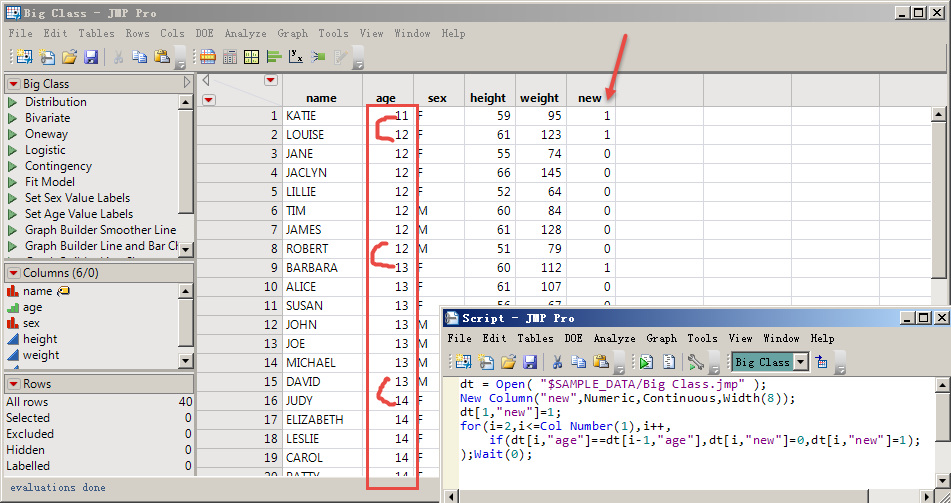Our World Statistics Day conversations have been a great reminder of how much statistics can inform our lives. Do you have an example of how statistics has made a difference in your life? Share your story with the Community!
Choose Language Hide Translation Bar
Highlighted

## Is there a more efficient way to do this in JSL than in a loop?

After sorting jm by the age column, add a new column, find out the age of the adjacent rows up and down the age is not returned at the same time 1.I try to cycle and feel slow myself.Ask for better methods.Thank you very much!

dt = Open( "\$SAMPLE_DATA/Big Class.jmp" );
New Column("new",Numeric,Continuous,Width(8));
dt[1,"age"]=1;
for(i=1,i<=Col Number(1),i++,
if(dt[i,"age"]==dt[i-1,"age"],dt[i,"new"]=0,dt[i,"new"]=1);
);2 REPLIES 2
Highlighted

## Re: Is there a more efficient way to do this in JSL than in a loop?

Note, the script you pasted does not match the script in the screen shot.

``````Names Default to Here(1);
dt = Open( "\$SAMPLE_DATA/Big Class.jmp" );

dt << Sort( By( :age ), Order( Ascending ), Replace Table );

dt << New Column("new",Numeric,Continuous,Width(8));
t0 = hptime();
dt[1,"new"]=1;

for(i=2,i<=Col Number(1),i++,
if(dt[i,"age"]==dt[i-1,"age"],dt[i,"new"]=0,dt[i,"new"]=1);
);
t1 = hptime();

dt << New Column("new2",Numeric,Continuous,Width(8));
t2 = hptime();
column(dt, "new2") << set each value(
If(row() == 1,  1,
:age == Lag(:age,1), 0,
//else
1)
);
t3 = hptime();

show(t3-t2, t1-t0);  //The results will be different each time, but the second method takes less than 50% of the time //compared to the for loop.//t3 - t2 = 257;  t1 - t0 = 679;``````

Highlighted

## Re: Is there a more efficient way to do this in JSL than in a loop?

Thank you very much!I'm going to use the example comparison later.
Article Labels

There are no labels assigned to this post.